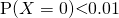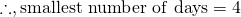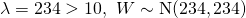# 2013 A-level H2 Mathematics (9740) Paper 2 Question 12 Suggested SolutionsAll solutions here are SUGGESTED. Mr. Teng will hold no liability for any errors. Comments are entirely personal opinions.

(i)
Conditions:
1. The mean number of absentees per unit time is constant.
2. The probability of an employee being absent is independent of another employee being absent.

Reasons why condition might not be met
1. The mean number of absentees may be higher during an epidemic.
2. If an employee is ill, his illness may be contagious and will increase the probability of other employees becoming ill.

(ii)
Let X denote the number of absentees from Admin Department on n days.(iii)
Let Y denote the number of days of absence from the 2 departments in 5 days.(iv)
Let W denote the number of days of absence from the 2 departments in 60 days.Sinceapproximately.by continuity correctionStudents need to take note that question must be answered in the context of the question. Most A-levels questions mandate this, so students should not just simply memorise the textbook answers. Some students do not realised that theformula for a poisson distribution is given in MF15 and could use it. Alternatively, students could have used the GC and draw the table in their answers. Lastly, do remember to apply continuity correction whenever necessary.
Not readable? Change text.